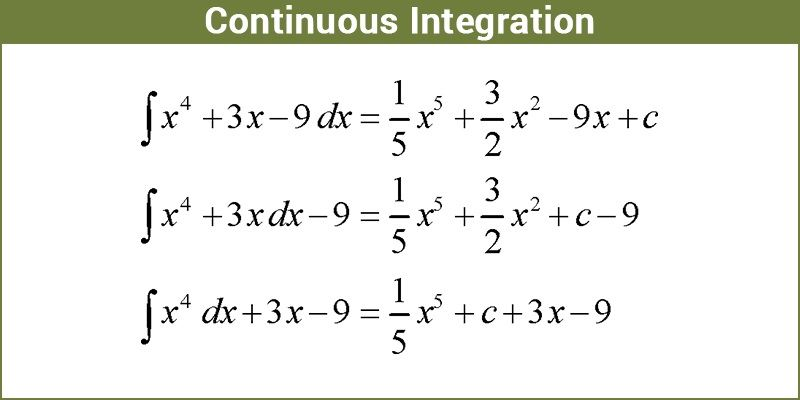In Maths, continuous integration is actually the designating of actual numbers to some functions that have some potential for negligible data or value for it. The continuous integral value is usually useful for expressing displacement, volume, area, and other dimensions of mathematics. By bringing together minuscule data integrals assign a number to a function in such a manner that describes volume, area and displacement. The two major operations of calculus are integration and differentiation.

Given a function f of a real variable x and an interval [a, b] of the real line, the definite integral.## What is Continuous Integration?

The connection between differential and integral calculus is through the fundamental theorem of calculus. In particular, if f(x) is continuous in the interval a <= x <=b and G(x) is a function such that (dG)/(dx) = f(x) for all values of x in (a, b), then

Let f be continuous on an interval I. Choose a point a in I. Then the function F(x) defined by

$$\begin{array}{l}\large F(x)=\int_{a}^{x}f(t)\;dt\end{array}$$

Let c be in I, and let x be infinitely close to c and between the endpoints of I. By the Addition Property,

$$\begin{array}{l}\large \int_{a}^{c}f(t)\;dt=\int_{a}^{x}f(t)\;dt+\int_{x}^{c}f(t)\;dt,\end{array}$$

$$\begin{array}{l}\large \int_{a}^{c}f(t)\;dt-\int_{a}^{x}f(t)\;dt=\int_{x}^{c}f(t)\;dt,\end{array}$$

$$\begin{array}{l}\large f(c)-F(x)=\int_{x}^{c}f(t)\;dt.\end{array}$$

Example to find out is continuous integration:

In what is continuous integration, let f(y) = in y, u(α) = a and v(α)=α

In this case, the function doesn’t depend on α Consequently, it has to be substitute with u, v, and f.

$$\begin{array}{l}\frac{(d)}{(d \alpha)}\int_a^{\alpha}\;In,y,dy= \frac{(f\alpha)(d, a)}{(d\;\alpha)}\end{array}$$

### Types of Integration

Integration has the estimate of a definite integral in general mathematics, the basic theory of calculus that allows among many other applications for initially computing the broad areas and actual averaging of the continuous functions.

Another type in integration is Numerical Integration. This integration is one amongst the numerical approaches for the evaluation and computation typically with computer operations, definite integrals and generally, solutions of differential equations.

One more subtype is the Order of integration. The number of times a time scale decreases for the sole objective of getting it fixed is explained by it. Indefinite integration, on the other hand, is the method of computing indefinite integrals which are basically called anti-derivatives, in calculus.